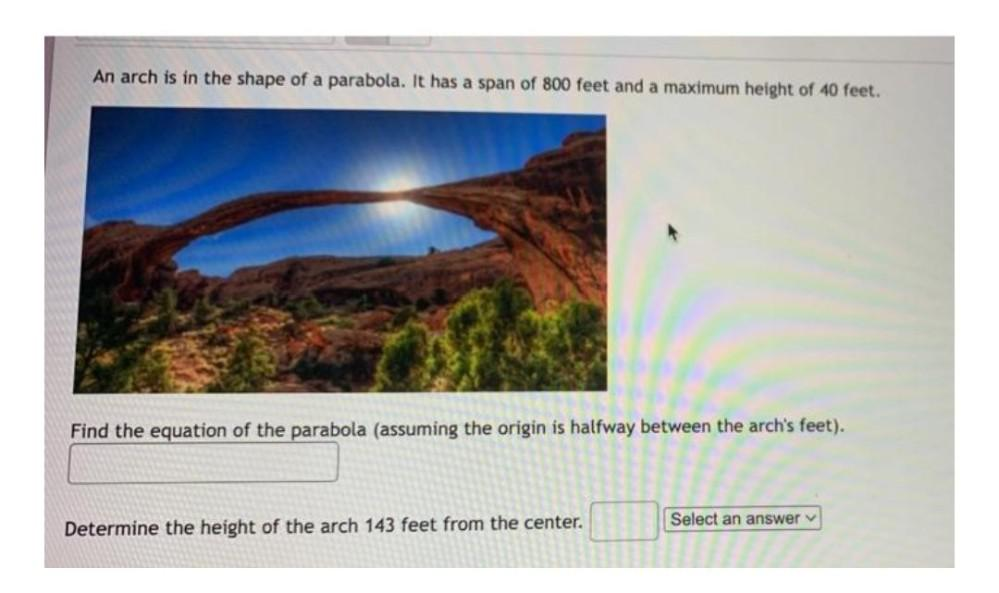Question:

# An arch is in the shape of a parabola. It has a span of 800 feet and a maximum height of 40 feet. Find the equation of the parabola (assuming the origin is halfway between the arch's feet).Determine tAn arch is in the shape of a parabola. It has a span of 800 feet and a maximum height of 40 feet. Find the equation of the parabola (assuming the origin is halfway between the arch's feet).Determine the height of the arch 143 feet from the center.